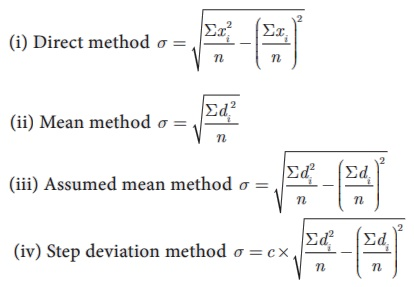Home | | Maths 10th Std | Points to Remember

# Points to Remember

Mathematics : Statistics And Probability: Points to Remember

Points to Remember

·           Range = L–S (L - Largest value, S - Smallest value)

·           Coefficient of range =; Variance·           Standard deviation·           Standard deviation (ungrouped data)·           Standard deviation of first n natural numbers·           Standard deviation (grouped data)·           Coefficient of variation C.V =× 100%

·           If the C.V. value is less, then the observations of corresponding data are consistent.

If the C.V. value is more then the observations of corresponding are inconsistent.

·           In a random experiment, the set of all outcomes are known but exact outcome is not known.

·           The set of all possible outcomes is called sample space.

·           A, B are said to be mutually exclusive events if A ∩ B = ɸ

·           Probability of event E is P (E) = n(E)/n(S)

(i) The probability of sure event is 1 and the probability of impossible event is 0.

(ii) 0 ≤ P (E) ≤ 1 ;(iii) P () = 1 − P (E)

·           If A and B are mutually exclusive events then P (A B ) = P(A) + P(B) .

(i)  P (A ∩) = P (onlyA) = P (A) – P (A ∩ B)

(ii) P (A ∩) = P (onlyB) = P (B) – P (A ∩ B)

·           P (A U B) = P (A) + P (B) – P (A ∩ B) , for any two events A, B.

·           For any three events A, B, C

P (A U B UC) = P (A) + P (B) + P (C) − P (A ∩ B ) − P(B ∩C) −P (C ∩ A) + P(A ∩ B ∩C)

Tags : Statistics and Probability | Mathematics , 10th Mathematics : UNIT 8 : Statistics And Probability
Study Material, Lecturing Notes, Assignment, Reference, Wiki description explanation, brief detail
10th Mathematics : UNIT 8 : Statistics And Probability : Points to Remember | Statistics and Probability | Mathematics